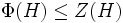# Weakly critical subgroup

BEWARE! This term is nonstandard and is being used locally within the wiki. [SHOW MORE]
This article defines a subgroup property: a property that can be evaluated to true/false given a group and a subgroup thereof, invariant under subgroup equivalence. View a complete list of subgroup properties[SHOW MORE]

## Definition

### Symbol-free definition

A subgroup of a group of prime power order (or more generally, of a possibly infinite p-group) is termed a critical subgroup if it satisfies the following three conditions:

1. The subgroup is a Frattini-in-center group: Its Frattini subgroup is contained in its center.
2. The subgroup is a commutator-in-center subgroup: Its commutator with the whole group is contained in its center.
3. The subgroup is a self-centralizing subgroup: Its centralizer in the whole group is contained in it.

### Definition with symbols

Let$G$ be a group of prime power order (or more generally, a possibly infinite p-group).

A subgroup$H$ of$G$ is said to be critical if the following three conditions hold:

1.$\Phi(H) \le Z(H)$, i.e., the Frattini subgroup is contained inside the center (i.e.,$H$ is a Frattini-in-center group).
2.$[G,H] \le Z(H)$ (i.e.,$H$ is a commutator-in-center subgroup of$G$).
3.$C_G(H)= Z(H)$ (i.e.,$H$ is a self-centralizing subgroup of$G$).

## Metaproperties

### Intermediate subgroup condition

YES: This subgroup property satisfies the intermediate subgroup condition: if a subgroup has the property in the whole group, it has the property in every intermediate subgroup.
ABOUT THIS PROPERTY: View variations of this property satisfying intermediate subgroup condition | View variations of this property not satisfying intermediate subgroup condition
ABOUT INTERMEDIATE SUBROUP CONDITION:View all properties satisfying intermediate subgroup condition | View facts about intermediate subgroup condition

If$H \le K \le G$ such that$H$ is weakly critical in$G$, then$H$ is weakly critical in$K$.